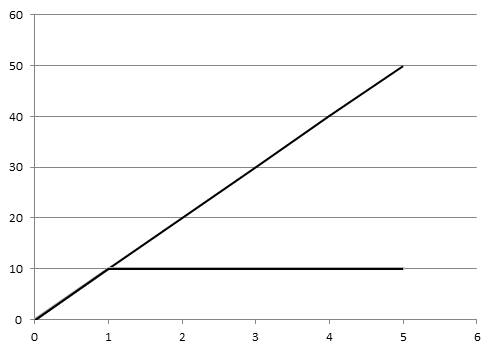# Derivation of TR, AR and MR curves under the perfect competition market

Derivation of TR, AR and MR curves under the perfect competition market:

Under the perfect competition market there are large no. of buyers and sellers. Producers are homogeneous. Market price is determined by market forces i.e. demand and supply. So, any individual consumer and seller can’t influence in the market price. Price of any particular commodity remains constant everywhere in an economy. On the basis of this concept, by the help of given table we can derive TR, AR and MR curves.

 Output Sold (Q) Price (P) TR = P × Q AR = TR / Q MR = ∆TR / ∆Q 1 10 10 10 10 2 10 20 10 10 3 10 30 10 10 4 10 40 10 10 5 10 50 10 10

On the above table, when output sold increases at equal rate, price remains constant. We calculate average, total and marginal revenue accordingly using formula. After calculation, we can observe that TR increases at the equal rate but AR and MR remains constant during the production period.

On the basis of given table, we can derive figure as:On the above figure, output sold is on x-axis and TR, AR, MR is on y-axis. With the increase in sale output increases at constant rate so TR slope is positively sloped but AR abs MR are equal with price with any quantity of output sold so that both are coincided with each other and parallel to x-axis.